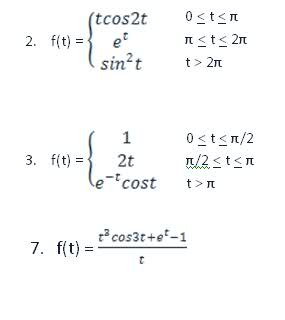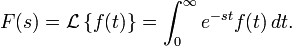Laplace Transform

Homework StatementHomework EquationsThe Attempt at a Solution

I don't know if it is possible to use Derivative of a transform while it is on unit step function procedure.

How can these questions be solved? Thank you.

The Attempt at a Solution

You just need to do some ugly integrals. What's the problem?

If you want to do it using step functions or indicator functions or whatever your field calls them, write f(t) in terms of them, it doesn't really simplify the problem, just shows a preference in notation. For example,

If $f(t)=\begin{cases} a(t), & t\in[0,a)\\ b(t), & t\in[a,\infty) \end{cases}$

f(t) can also be written in terms of the indicator functions as follows,

$f(t)=a(t)\chi_{t\in[0,a)}+b(t)\chi_{t\in[a,\infty)}$.

The change of notation in terms of step functions is nothing special, the thing that is really relevant is what happens when you integrate over them from 0 to infinity. What you should get is more than one integral.

I don't know if this was your question, or if you need help doing the integrals.

You can simplify the procedure slightly if you note that the Laplace transformation of exp(at) for any a that's a real number times a hard function you already know the Laplace transform of is just shifted. Maybe that helps more.

Last edited: